# simtrait: Simulate Complex Traits from Genotypes

#### 2021-08-12

$$\DeclareMathOperator{\E}{E}$$ $$\DeclareMathOperator{\Cov}{Cov}$$

# Introduction

This vignette has three main parts:

1. Practical examples that show how to use the functions and demonstrate that the random traits generated by this package have the desired covariance structure.

2. The mathematical trait model that motivated this package.

3. The algorithm implementation, which follows straightforwardly from the model when ancestral allele frequencies are known. However, more painful details are necessary when ancestral allele frequencies must be estimated from the genotypes, which induces biases that fortunately can be corrected.

# Sample usage

In this section we first simulated an admixed population using bnpsd, then we simulate traits using simtrait. In particular, we simulate a large number of traits to demonstrate that their sample covariance matrix is as expected.

## Load libraries required for this vignette

library(popkin)   # to create plots of our covariance matrices
library(bnpsd)    # to simulate an admixed population
library(simtrait) # this package

# dimensions of data/model
# number of loci
m_loci <- 10000
# number of individuals, smaller than usual for easier visualizations
n_ind <- 30
# number of intermediate subpops (source populations for admixed individuals)
k_subpops <- 3

# define population structure
# FST values for 3 subpopulations (proportional/unnormalized)
inbr_subpops <- 1 : k_subpops
bias_coeff <- 0.5 # bias coeff of standard Fst estimator
Fst <- 0.3 # desired final Fst
n_ind = n_ind,
k_subpops = k_subpops,
bias_coeff = bias_coeff,
coanc_subpops = inbr_subpops,
fst = Fst
)
admix_proportions <- obj$admix_proportions # rescaled Fst vector for intermediate subpops inbr_subpops <- obj$coanc_subpops

# get pop structure parameters of the admixed individuals
kinship <- coanc_to_kinship(coancestry)

# draw allele freqs and genotypes
X <- out$X # genotypes p_anc <- out$p_anc # ancestral AFs

## Simulate a trait from random coefficients (RC) model

First we simulate one trait. Note that we pick non-default values for the mean mu and variance factor sigma_sq to validate that these can be anything we want.

# parameters of simulation
m_causal <- 100
herit <- 0.8
# default 0, let's try a non-trivial case
mu <- 1
# default 1, also let's see that this more complicated case works well
sigma_sq <- 1.5

# create simulated trait
# case of exact p_anc
obj <- sim_trait(
X = X,
m_causal = m_causal,
herit = herit,
p_anc = p_anc,
mu = mu,
sigma_sq = sigma_sq
)
# trait vector
length(obj$trait) #>  30 n_ind #>  30 obj$trait
#>   -0.31548046  0.65829722 -0.63858118  0.81983900  0.28945527  0.21907610
#>    1.51318147  0.78172249 -0.72533482  0.65195700  1.05774192 -0.52168851
#>   0.04655130  0.81555908  2.11404738  0.24768786  1.90588945  2.38728156
#>   0.45706915  0.86774285 -0.22793418  0.35222720  0.49109987 -1.44563652
#>   0.63525500  0.06257298  0.17634807  0.35173647  0.31411011  0.50555147
# randomly-picked causal locus indexes
length( obj$causal_indexes ) #>  100 m_causal #>  100 head( obj$causal_indexes ) # show partially...
#>  1593 7240 3066 1001 9050 2821
# regression coefficients vector
length( obj$causal_coeffs ) #>  100 m_causal #>  100 head( obj$causal_coeffs ) # show partially...
#>  -0.09104072 -0.03886332  0.17225609 -0.04928302  0.04779169 -0.18103885

## Compare sample covariance of trait to theoretical expectation

The interesting validation is simulation a large number of random traits, from which we can estimate a sample covariance matrix to compare to the desired theoretical one. We shall compare this to other versions of the simulation. We distinguish this version as having random coefficients (RC) and employing true allele frequencies p_anc.

# the theoretical covariance matrix of the trait is calculated by cov_trait
V <- cov_trait(kinship = kinship, herit = herit, sigma_sq = sigma_sq)

# simulate these many traits
n_traits <- 1000
# store in this matrix, initialize with zeroes
Y_rc_freq <- matrix(data = 0, nrow = n_traits, ncol = n_ind)
# start loop
for (i in 1 : n_traits) {
obj <- sim_trait(
X = X,
m_causal = m_causal,
herit = herit,
p_anc = p_anc,
mu = mu,
sigma_sq = sigma_sq
)
Y_rc_freq[i,] <- obj$trait # store in i^th row } # estimate sample covariance V_rc_freq <- cov(Y_rc_freq) First let’s verify that the mean is as expected. Below the red line marks the desired mean. par_orig <- par(mgp = c(2, 0.5, 0)) # reduce margins from default par(mar = c(3.5, 3, 0, 0) + 0.2) # visualize distribution boxplot( list( 'RC freq' = rowMeans(Y_rc_freq) ), xlab = "Trait Type", ylab = 'Sample Mean' ) # red line marks expected mean abline(h = mu, col = 'red')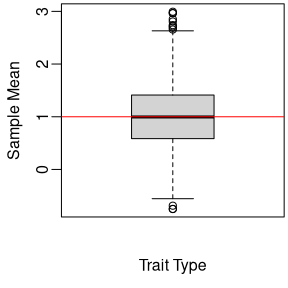par( par_orig ) # reset par Now let’s visualize the covariance matrices using plot_popkin from the popkin package. Since both matrices have large diagonals, we shrink them somewhat using inbr_diag also from the popkin package. plot_popkin( inbr_diag(list(V, V_rc_freq)), titles = c('Theoretical', 'RC freq'), leg_title = 'Covariance', # set margin for title (top is non-zero) mar = c(0, 2) )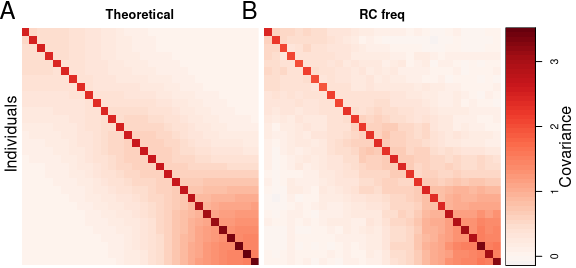This plot verifies that the empirical covariance matches the theoretical expectation! ## Simulated trait without ancestral allele frequencies For real data, true ancestral allele frequencies are unknown. A reasonable trait can still be simulated in these cases, but this solution no longer has theoretical guarantees to yield the desired mean value in particular. This solution relies on a known mean kinship to compensate for the biases of estimated ancestral allele frequencies. A good kinship matrix estimate can be obtained using the popkin package. For simplicity, here we use the true kinship matrix rather than an estimate: # store this in new matrix Y_rc_kin <- matrix(data = 0, nrow = n_traits, ncol = n_ind) # start loop for (i in 1 : n_traits) { obj <- sim_trait( X = X, m_causal = m_causal, herit = herit, # whole kinship matrix can be passed instead of just mean kinship = kinship, mu = mu, sigma_sq = sigma_sq ) Y_rc_kin[i,] <- obj$trait # store in i^th row
}
# estimate sample covariance
V_rc_kin <- cov(Y_rc_kin)

First let’s verify the means again. Recall the red line marks the desired mean. Below the original sample (simulated using the true p_anc) is shown first as “RC freq”, while the new sample based on the kinship matrix is “RC kinship”:

par_orig <- par(mgp = c(2, 0.5, 0))
# reduce margins from default
par(mar = c(3.5, 3, 0, 0) + 0.2)
# visualize distribution
boxplot(
list(
"RC freq" = rowMeans(Y_rc_freq),
"RC kinship" = rowMeans(Y_rc_kin)
),
xlab = "Trait Type",
ylab = 'Sample Mean'
)
# red line marks expected mean
abline(h = mu, col = 'red')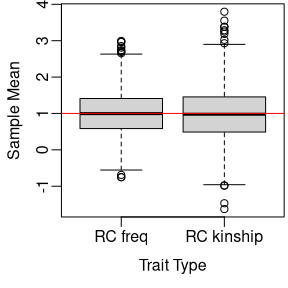par( par_orig ) # reset par

Now we compare all three matrices:

plot_popkin(
inbr_diag(list(V, V_rc_freq, V_rc_kin)),
titles = c('Theoretical', 'RC freq', 'RC kinship'),
leg_title = 'Covariance',
mar = c(0, 2)
)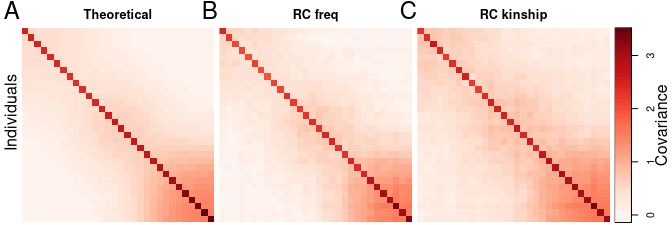This plot shows again good agreement between the sample covariance matrix of traits simulated without true ancestral allele frequencies (“RC kinship”) and the desired “theoretical” covariance matrix.

## Simulated trait from infinitesimal model

An alternative approach for simulating traits is by drawing them from a Multivariate Normal (MVN) model with the desired mean and covariance structure. This is often called the infinitesimal model, since it follows from the central limit theorem under the assumption that there are infinite causal loci, each with an infinitesimal effect size. A trait simulated this way has no use in GWAS tests, as there are no causal loci (in other words, the null hypothesis holds across the genome). However, these traits have a heritability that can be estimated, and in fact this infinitesimal model is assumed by approaches that estimate heritability by fitting variance components, such as GCTA (Yang et al. 2011).

We draw the MVN traits this way:

# This function simulates trait replicates in one call,
# generating a matrix comparable to the previous ones.
Y_mvn <- sim_trait_mvn(
rep = n_traits,
kinship = kinship,
herit = herit,
mu = mu,
sigma_sq = sigma_sq
)
# estimate sample covariance
V_mvn <- cov(Y_mvn)

First let’s verify the means again. Recall the red line marks the desired mean. The new sample is denoted as “MVN”, and the other two are as in the previous sections (traits simulated from genotypes, using the true p_anc or with bias corrections from the kinship matrix):

par_orig <- par(mgp = c(2, 0.5, 0))
# reduce margins from default
par(mar = c(3.5, 3, 0, 0) + 0.2)
# visualize distribution
boxplot(
list(
"RC freq" = rowMeans(Y_rc_freq),
"RC kinship" = rowMeans(Y_rc_kin),
"MVN" = rowMeans(Y_mvn)
),
xlab = "Trait Type",
ylab = 'Sample Mean'
)
# red line marks expected mean
abline(h = mu, col = 'red')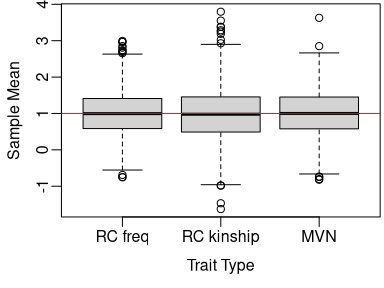par( par_orig ) # reset par

Now we compare all four covariance matrices:

plot_popkin(
inbr_diag(list(V, V_rc_freq, V_rc_kin, V_mvn)),
titles = c('Theoretical', 'RC freq', 'RC kinship', 'MVN'),
leg_title = 'Covariance',
mar = c(0, 2),
leg_width = 0.4
)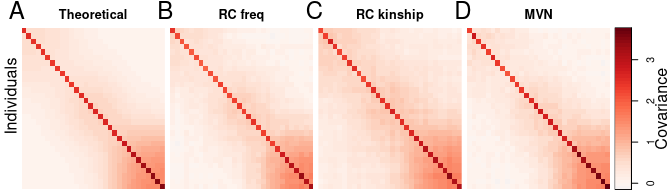This plot shows again good agreement between the sample covariance matrix of traits simulated under the infinitesimal model (“MVN”) and the desired “theoretical” covariance matrix and first two simulations.

## Simulated trait from fixed effect sizes (FES) model

This package can also simulate traits from a model where coefficients are larger for rarer variants, which may be more realistic for disease traits where selection prevents common variants from having large coefficients, while allowing rare variants to have larger coefficients. The effect size of locus $$i$$ is its variance contribution, equal to $$2 \beta^2_i p_i(1-p_i)$$ for outbred individuals, where $$\beta_i$$ is the regression coefficient and $$p_i$$ is the ancestral allele frequency. The limit of strong negative and other modes of selection forces effect sizes to be equal for all loci, so the coefficient at locus $$i$$ is proportional to $$1 / \sqrt{p_i(1-p_i)}$$. As in the other models, the coefficients are rescaled to yield the desired heritability and variance factor. This is related to previous models proposed in the literature, for example (Speed et al. 2012).

This time we simulate both the true allele frequency version, “freq”, and the “kinship” version that unbiases sample allele frequencies.

# store this in new matrix
Y_fes_freq <- matrix(data = 0, nrow = n_traits, ncol = n_ind)
Y_fes_kin <- matrix(data = 0, nrow = n_traits, ncol = n_ind)
# start loop
for (i in 1 : n_traits) {
obj <- sim_trait(
X = X,
m_causal = m_causal,
herit = herit,
p_anc = p_anc,
mu = mu,
sigma_sq = sigma_sq,
fes = TRUE # only diff from orig run
)
Y_fes_freq[i,] <- obj$trait # store in i^th row obj <- sim_trait( X = X, m_causal = m_causal, herit = herit, kinship = kinship, mu = mu, sigma_sq = sigma_sq, fes = TRUE # only diff from orig run ) Y_fes_kin[i,] <- obj$trait # store in i^th row
}
# estimate sample covariance
V_fes_freq <- cov(Y_fes_freq)
V_fes_kin <- cov(Y_fes_kin)

First let’s verify the means again. Recall the red line marks the desired mean. The new samples are labeled as “FES freq” and “FES kinship”, and the first three are as in the previous sections (traits simulated from genotypes and the random coefficients (RC) model, using the true p_anc or with bias corrections from the kinship matrix, and the MVN traits):

par_orig <- par(mgp = c(2, 0.5, 0))
# reduce margins from default
par(mar = c(3.5, 3, 0, 0) + 0.2)
# visualize distribution
boxplot(
list(
"RC freq" = rowMeans(Y_rc_freq),
"RC kinship" = rowMeans(Y_rc_kin),
"MVN" = rowMeans(Y_mvn),
"FES freq" = rowMeans(Y_fes_freq),
"FES kinship" = rowMeans(Y_fes_kin)
),
xlab = "Trait Type",
ylab = 'Sample Mean'
)
# red line marks expected mean
abline(h = mu, col = 'red')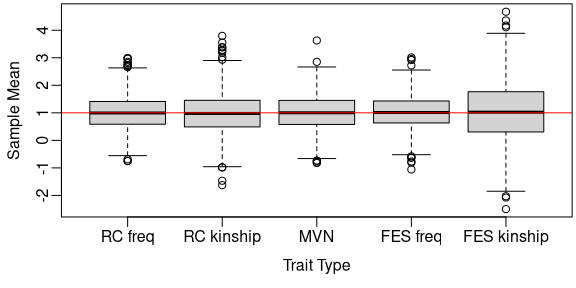par( par_orig ) # reset par

Now we compare all covariance matrices:

plot_popkin(
inbr_diag( list( V, V_rc_freq, V_rc_kin, V_mvn, V_fes_freq, V_fes_kin ) ),
titles = c('Theoretical', 'RC freq', 'RC kinship', 'MVN', 'FES freq', 'FES kinship'),
leg_title = 'Covariance',
mar = c(0, 2),
leg_width = 0.4,
layout_rows = 2
)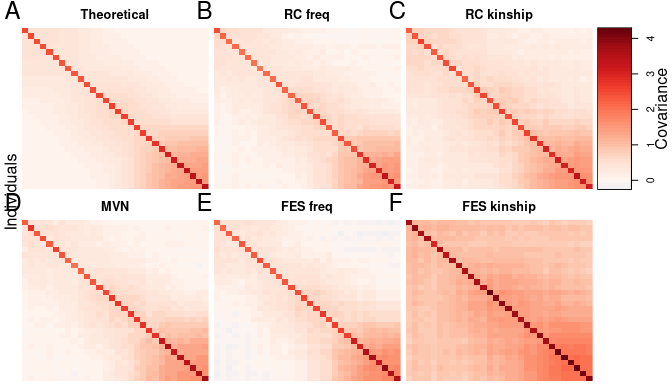This plot shows again good agreement between the sample covariance matrix of traits simulated under this “fixed effect sizes” model (“FES freq” and “FES kinship”) and the desired “theoretical” covariance matrix and first three simulations.

# Model

Here is a brief summary of the trait model, which explains what this package does internally.

Suppose there are $$n$$ individuals and $$m$$ (causal) loci. For simplicity we shall assume that every locus has a regression coefficient, although in practice many of these coefficients will be zero. The following variables are part of the model:

Variable Dimensions Description
$$\mathbf{X}$$ $$n \times m$$ Genotypes
$$\mathbf{x}_i$$ $$n \times 1$$ Genotype vector at locus $$i$$
$$\mathbf{y}$$ $$n \times 1$$ Trait
$$\mathbf{\beta}$$ $$m \times 1$$ Regression coefficients
$$\mathbf{\epsilon}$$ $$n \times 1$$ Non-genetic effects
$$\mathbf{p}$$ $$m \times 1$$ Ancestral allele frequencies
$$\mathbf{\Phi}$$ $$n \times n$$ Kinship matrix
$$\alpha$$ $$1 \times 1$$ Intercept coefficient
$$\mu$$ $$1 \times 1$$ Trait mean
$$h^2$$ $$1 \times 1$$ Heritability
$$\sigma^2$$ $$1 \times 1$$ Trait variance factor
$$\mathbf{1}$$ $$n \times 1$$ Vector of ones
$$\mathbf{0}$$ $$n \times 1$$ Vector of zeroes
$$\mathbf{I}$$ $$n \times n$$ Identity matrix

We assume the linear polygenic model for a quantitative trait: $\mathbf{y} = \alpha \mathbf{1} + \mathbf{X} \mathbf{\beta} + \mathbf{\epsilon}.$ To analyze the covariance structure of the trait, we shall assume that $$\alpha$$ and $$\mathbf{\beta}$$ are fixed parameters, while $$\mathbf{X} = (\mathbf{x}_i)$$ and $$\mathbf{\epsilon}$$ are random with expectations and covariances of \begin{align*} \E[\mathbf{X}] &= 2 \mathbf{1} \mathbf{p}^\intercal , \\ \Cov(\mathbf{x}_i) &= 4 p_i (1-p_i) \mathbf{\Phi} , \\ \E[\mathbf{\epsilon}] &= \mathbf{0} , \\ \Cov(\mathbf{\epsilon}) &= (1-h^2) \sigma^2 \mathbf{I} , \end{align*} where $$\mathbf{p} = (p_i)$$. The expectation of the trait is therefore \begin{align*} \E[\mathbf{y}] &= \alpha \mathbf{1} + \E[\mathbf{X}] \mathbf{\beta} + \E[\mathbf{\epsilon}] \\ &= \alpha \mathbf{1} + 2 \mathbf{1} \mathbf{p}^\intercal \mathbf{\beta} , \end{align*} which can be written as \begin{align*} \E[\mathbf{y}] = \mu \mathbf{1} , \quad \text{where} \quad \mu = \alpha + 2 \mathbf{p}^\intercal \mathbf{\beta} . \end{align*} The covariance matrix of the trait is \begin{align*} \Cov(\mathbf{y}) &= \sum_{i=1}^m \Cov(\mathbf{x}_i) \beta_i^2 + \Cov(\mathbf{\epsilon}) \\ &= \mathbf{\Phi} \sum_{i=1}^m 4 p_i (1-p_i) \beta_i^2 + (1-h^2) \sigma^2 \mathbf{I} , \end{align*} where $$\mathbf{\beta} = (\beta_i)$$. Therefore, we can write the covariance in terms of the heritability and the overall variance scale: \begin{align*} \Cov(\mathbf{y}) &= \sigma^2 \left( 2 h^2 \mathbf{\Phi} + (1-h^2) \mathbf{I} \right) , \quad \text{where} \\ \sigma^2 h^2 &= \sum_{i=1}^m 2 p_i (1-p_i) \beta_i^2 . \end{align*} The factor of two in front of $$\mathbf{\Phi}$$ is traditionally there since for an unstructured population $$2 \mathbf{\Phi} = \mathbf{I}$$, in which case the trait covariance simplifies to $$\Cov(\mathbf{y}) = \sigma^2 \mathbf{I}$$ for any value of $$h^2$$. More broadly, the variance of the trait for any outbred individual is $$\sigma^2$$ under this parametrization.

# Algorithm

In all cases the user sets the heritability and other parameters but not the coefficients $$\mathbf{\beta}$$ directly. To choose $$\mathbf{\beta}$$, the algorithm initially draws random coefficients or sets them using a formula (depending on the model) and scales them to yield the desired covariance structure.

The user provides a genotype matrix and sets the number of causal loci. The algorithm selects random loci to be the causal ones. From this moment on $$\mathbf{X}$$ will contain only those causal loci.

## Constructing coefficients

Under the random coefficients (RC) model, the initial coefficients are drawn independently from a standard normal distribution: $\beta_i \sim \text{N}(0,1).$

Under the fixed effect sizes (FES) model, the initial coefficients are $\beta_i = 1 / \sqrt{p_i(1-p_i)}.$ (When $$p_i$$ are unknown, their sample estimates are used for this step.) Lastly, the sign of $$\beta_i$$ is drawn randomly (it is negative with probability 0.5).

Again, whichever form these coefficients take, they are rescaled to result in the desired heritability and variance factor using the procedures described next.

Below we divide the algorithm into two steps: (1) scaling the coefficients, and (2) centering the trait. Each step forks into two cases: whether the true ancestral allele frequencies are known or not (the latter requires a known mean kinship).

## Scaling coefficients

### Scaling using known ancestral allele frequencies

Here we assume that $$\mathbf{p} = (p_i)$$ is provided by the user. The user has also provided the desired values of both $$h^2$$ and $$\sigma^2$$. The initial genetic variance factor is $\sigma^2_0 = \sum_{i=1}^m 2 p_i (1-p_i) \beta_i^2.$ We obtain the desired variance by dividing each $$\beta_i$$ by $$\sigma_0$$ (which results in a variance of 1) and then multiply by $$h \sigma$$ (which finally results in the desired variance of $$h^2 \sigma^2$$). Combining both steps, the update is $\mathbf{\beta} \leftarrow \mathbf{\beta} \frac{ h \sigma }{\sigma_0}.$

### Scaling using a known kinship matrix

When $$\mathbf{p}$$ isn’t known, sample estimates $$\mathbf{\hat{p}}$$ are constructed from the genotype data. Let $\hat{p}_i = \frac{1}{2n} \mathbf{1}^\intercal \mathbf{x}_i .$ Although this estimator is unbiased ($$\E[\mathbf{\hat{p}}] = \mathbf{p}$$), the resulting variance estimates of interest are downwardly biased (Ochoa and Storey 2016): $\E \left[ \hat{p}_i \left( 1-\hat{p}_i \right) \right] = p_i(1-p_i) (1 - \bar{\varphi}),$ where $$\bar{\varphi} = \frac{1}{n^2} \mathbf{1}^\intercal \mathbf{\Phi} \mathbf{1}$$ is the mean kinship coefficient in the data. Therefore the initial genetic variance factor, estimated as $\hat{\sigma}^2_0 = \sum_{i=1}^m 2 \hat{p}_i (1-\hat{p}_i) \beta_i^2,$ has an expectation of $\E \left[ \hat{\sigma}^2_0 \right] = \sigma^2_0 (1 - \bar{\varphi})$ Since this additional factor $$(1 - \bar{\varphi})$$ is known in this setting, the adjusted update $\mathbf{\beta} \leftarrow \mathbf{\beta} \frac{ h \sigma \sqrt{1-\bar{\varphi}} }{\hat{\sigma}_0}$ also results in the desired variance.

## Centering the trait

### Centering using known ancestral allele frequencies

This is the preferred approach as it is the only case that guarantees success. Given our model, we obtain the desired overall trait mean $$\mu$$ by choosing the intercept to be $\alpha = \mu - 2 \mathbf{p}^\intercal \mathbf{\beta}$

### Centering without ancestral allele frequencies

The solution that this version of the algorithm takes is to choose the intercept \begin{align*} \alpha &= \mu - 2 \hat{\bar{p}} \mathbf{1}_m^\intercal \mathbf{\beta} , \quad \text{where} \\ \hat{\bar{p}} &= \frac{1}{m} \mathbf{1}_m^\intercal \mathbf{\hat{p}} = \frac{1}{2 m n} \mathbf{1}_m^\intercal \mathbf{X}^\intercal \mathbf{1}_n = \frac{1}{2} \bar{X} , \end{align*} where $$\mathbf{1}_m$$ above are length-$$m$$ vectors of ones. This works very well in practice since $$\mathbf{\beta}$$ is drawn randomly, so it is uncorrelated with the true $$\mathbf{p}$$ (this is true in FES too since the sign of each coefficient is random). In this setting it suffices to consider each coefficient $$\beta_i$$ as acting on the average locus, which is treated as having a random ancestral allele frequency $$p_i$$, and all that matters is the global mean of $$p_i$$ values.

### How NOT to center the trait vector

Now let’s discuss why the obvious way of centering the trait without known ancestral allele frequencies doesn’t work. Why not use the sample allele frequencies as $\alpha = \mu - 2 \mathbf{\hat{p}}^\intercal \mathbf{\beta} \quad ?$ Centering the trait this way is equivalent to centering genotypes at each locus: $\mathbf{y} = \alpha \mathbf{1} + \sum_{i=1}^m (\mathbf{x}_i - 2 \hat{p}_i \mathbf{1}) \beta_i + \mathbf{\epsilon}.$ However, this operation introduces a distortion in the covariance of the genotypes (Ochoa and Storey 2016): $\Cov \left( \mathbf{x}_i - 2 \hat{p}_i \mathbf{1} \right) = p_i (1-p_i) \left( \mathbf{\Phi} + \bar{\varphi} \mathbf{1}\mathbf{1}^\intercal - \mathbf{\varphi} \mathbf{1}^\intercal - \mathbf{1} \mathbf{\varphi}^\intercal \right),$ where $$\mathbf{\varphi} = \frac{1}{n} \mathbf{\Phi} \mathbf{1}$$. These undesirable distortions propagate to the trait, which we confirmed in simulations (not shown). It is not clear how these distortions can be corrected for after centering the trait as shown above.

Note that the intercept version we chose instead does not induce this genotype centering, which prevents the undesirable distortions in the trait covariance.